# NCERT Solutions for Class 9th: Ch 10 Circle Maths

#### NCERT Solutions for Class 9th: Ch 10 Circle Maths

Page No: 171

Exercise 10.1

1. Fill in the blanks:
(i) The centre of a circle lies in ____________ of the circle. (exterior/ interior)
(ii) A point, whose distance from the centre of a circle is greater than its radius lies in __________ of the circle. (exterior/ interior)
(iii) The longest chord of a circle is a _____________ of the circle.
(iv) An arc is a ___________ when its ends are the ends of a diameter.
(v) Segment of a circle is the region between an arc and _____________ of the circle.
(vi) A circle divides the plane, on which it lies, in _____________ parts.

(i)   The centre of a circle lies in interior of the circle. (exterior/interior)
(ii)  A point, whose distance from the centre of a circle is greater than its radius lies in exteriorof the circle. (exterior/interior)
(iii) The longest chord of a circle is a diameter of the circle.
(iv) An arc is a semicircle when its ends are the ends of a diameter.
(v) Segment of a circle is the region between an arc and chord of the circle.
(vi) A circle divides the plane, on which it lies, in three parts.

(i) Line segment joining the centre to any point on the circle is a radius of the circle.
(ii) A circle has only finite number of equal chords.
(iii) If a circle is divided into three equal arcs, each is a major arc.
(iv) A chord of a circle, which is twice as long as its radius, is a diameter of the circle.
(v) Sector is the region between the chord and its corresponding arc.
(vi) A circle is a plane figure.

(i) True.
All the line segment from the centre to the circle is of equal length.
(ii) False.
We can draw infinite numbers of equal chords.
(iii) False.
We get major and minor arcs for unequal arcs. So, for equal arcs on circle we can't say it is major arc or minor arc.
(iv) True.
A chord which is twice as long as radius must pass through the centre of the circle and is diameter to the circle.
(v) False.
Sector is the region between the arc and the two radii of the circle.
(vi) True.
A circle can be drawn on the plane.

Exercise 10.2

1. Recall that two circles are congruent if they have the same radii. Prove that equal chords of congruent circles subtend equal angles at their centres.

A circle is a collection of points whose every every point is equidistant from the centre. Thus, two circles can only be congruent when they the distance of every point of the both circle is equal from the centre.Given,
AB = CD (Equal chords)
To prove,
∠AOB = ∠COD
Proof,
In ΔAOB and ΔCOD,
AB = CD (Given)
∴ ΔAOB ≅ ΔCOD (SSS congruence condition)
Thus, ∠AOB = ∠COD by CPCT.
Equal chords of congruent circles subtend equal angles at their centres.

2. Prove that if chords of congruent circles subtend equal angles at their centres, then the chords are equal.Given,
∠AOB = ∠COD (Equal angles)
To prove,
AB = CD
Proof,
In ΔAOB and ΔCOD,
∠AOB = ∠COD (Given)
∴ ΔAOB ≅ ΔCOD (SAS congruence condition)
Thus, AB = CD by CPCT.
If chords of congruent circles subtend equal angles at their centres, then the chords are equal.

Page No: 176

Exercise 10.3

1. Draw different pairs of circles. How many points does each pair have in common? What is the maximum number of common points?No point is common.One point P is common.One point P is common.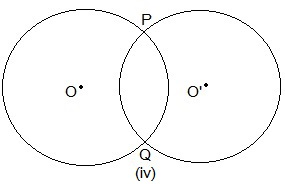Two points P and Q are common.No point is common.

2. Suppose you are given a circle. Give a construction to find its centre.Steps of construction:
Step I: A circle is drawn.
Step II: Two chords AB and CD are drawn.
Step III: Perpendicular bisector of the chords AB and CD are drawn.
Step IV: Let these two perpendicular bisector meet at a point. The point of intersection of these two perpendicular bisector is the centre of the circle.

3. If two circles intersect at two points, prove that their centres lie on the perpendicular bisector of the common chord.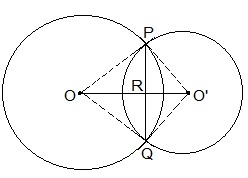Given,
Two circles which intersect each other at P and Q.
To prove,
OO' is perpendicular bisector of PQ.
Proof,
In ΔPOO' and ΔQOO',
OO' = OO' (Common)
∴ ΔPOO' ≅ ΔQOO' (SSS congruence condition)
Thus,
∠POO' = ∠QOO' --- (i)
In ΔPOR and ΔQOR,
∠POR = ∠QOR (from i)
OR = OR (Common)
∴ ΔPOR ≅ ΔQOR (SAS congruence condition)
Thus,
∠PRO = ∠QRO
also,
∠PRO + ∠QRO = 180°
⇒ ∠PRO = ∠QRO = 180°/2 = 90°
Hence,
OO' is perpendicular bisector of PQ.

Page No: 179

Exercise 10.4

1. Two circles of radii 5 cm and 3 cm intersect at two points and the distance between their centres is 4 cm. Find the length of the common chord.

OP = 5cm, PS = 3cm and OS = 4cm.
also, PQ = 2PR
Let RS be x.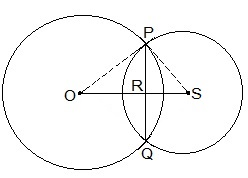In ΔPOR,
OP= OR+ PR2
⇒ 5= (4-x)+ PR2
⇒ 25 = 16 + x- 8x +  PR2
⇒ PR2 = 9 - x2 + 8x --- (i)

In ΔPRS,
PS= PR+ RS2
⇒ 3= PR2 + x2
⇒  PR2 = 9 - x2 --- (ii)

Equating (i) and (ii),
9 - x2 + 8x = 9 - x2
⇒ 8x = 0
⇒ x = 0
Putting the value of x in (i) we get,
PR2 = 9 - 02
⇒ PR = 3cm
Length of the cord PQ = 2PR = 2×3 = 6cm

2. If two equal chords of a circle intersect within the circle, prove that the segments of one chord are equal to corresponding segments of the other chord.

Given,
AB and CD are chords intersecting at E.
AB = CD
To prove,
AE = DE and CE = BE
Construction,
OM ⊥ AB and ON ⊥ CD. OE is joined.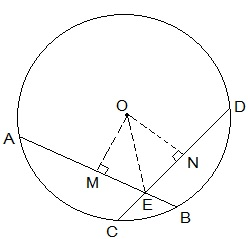Proof,
OM bisects AB (OM ⊥ AB)
ON bisects CD (ON ⊥ CD)
As AB = CD thus,
AM = ND --- (i)
and MB = CN --- (ii)
In ΔOME and ΔONE,
∠OME = ∠ONE (Perpendiculars)
OE = OE (Common)
OM = ON (AB = CD and thus equidistant from the centre)
ΔOME ≅ ΔONE by RHS congruence condition.
ME = EN by CPCT --- (iii)
From (i) and (ii) we get,
AM + ME = ND + EN
⇒ AE = ED
From (ii)  and (iii) we get,
MB - ME = CN - EN
⇒ EB = CE

3. If two equal chords of a circle intersect within the circle, prove that the line joining the point of intersection to the centre makes equal angles with the chords.

Given,
AB and CD are chords intersecting at E.
AB = CD, PQ is the diameter.
To prove,
∠BEQ = ∠CEQ
Construction,
OM ⊥ AB and ON ⊥ CD. OE is joined.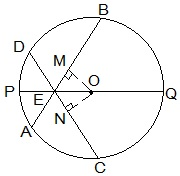In ΔOEM and ΔOEN,
OM = ON (Equal chords are equidistant from the centre)
OE = OE (Common)
∠OME = ∠ONE (Perpendicular)
ΔOEM ≅ ΔOEN by RHS congruence condition.
Thus,
∠MEO = ∠NEO by CPCT
⇒ ∠BEQ = ∠CEQ

4. If a line intersects two concentric circles (circles with the same centre) with centre O at A, B, C and D, prove that AB = CD (see Fig. 10.25).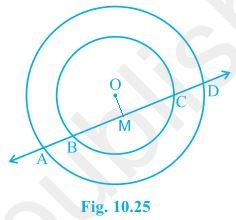OM ⊥ AD is drawn from O.
⇒ AM = MD --- (i)
also, OM bisects BC as OM ⊥ BC.
⇒ BM = MC --- (ii)
From (i) and (ii),
AM - BM = MD - MC
⇒ AB = CD

5. Three girls Reshma, Salma and Mandip are playing a game by standing on a circle of radius 5m drawn in a park. Reshma throws a ball to Salma, Salma to Mandip, Mandip to Reshma. If the distance between Reshma and Salma and between Salma and Mandip is 6m each, what is the distance between Reshma and Mandip?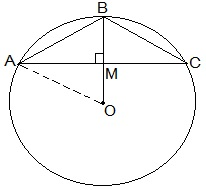Let A, B and C represent the positions of Reshma, Salma and Mandip respectively.
AB = 6cm and BC = 6cm.
BM ⊥ AC is drawn.
ABC is an isosceles triangle as AB = BC, M is mid-point of AC. BM is perpendicular bisector of AC and thus it passes through the centre of the circle.
Let AM = y and OM = x then BM = (5-x).
Applying Pythagoras theorem in ΔOAM,
OA= OM+ AM2
⇒ 5= x+ y--- (i)
Applying Pythagoras theorem in ΔAMB,
AB= BM+ AM2
⇒ 6= (5-x)+ y2 --- (ii)
Subtracting (i) from (ii), we get
36 - 25 = (5-x)- x2 -
⇒ 11 = 25 - 10x
⇒ 10x = 14 ⇒ x= 7/5
Substituting the value of x in (i), we get
y+ 49/25 = 25
⇒ y2 = 25 - 49/25
⇒ y2 = (625 - 49)/25
⇒ y2 = 576/25
⇒ y = 24/5
Thus,
AC = 2×AM = 2×y = 2×(24/5) m = 48/5 m = 9.6 m
Distance between Reshma and Mandip is 9.6 m.

6. A circular park of radius 20m is situated in a colony. Three boys Ankur, Syed and David are sitting at equal distance on its boundary each having a toy telephone in his hands to talk each other. Find the length of the string of each phone.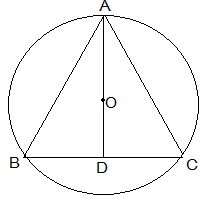Let A, B and C represent the positions of Ankur, Syed and David respectively. All three boys at equal distances thus ABC is an equilateral triangle.
AD ⊥ BC is drawn. Now, AD is median of ΔABC and it passes through the centre O.
Also, O is the centroid of the ΔABC. OA is the radius of the triangle.
Let the side of a triangle a metres then BD = a/2 m.
Applying Pythagoras theorem in ΔABD,
OA = 2/3 AD ⇒ 20 m = 2/3 × √3a/2
⇒ a = 20√3 m
Length of the string is 20√3 m.

Page No: 184

Exercise 10.5

1. In Fig. 10.36, A,B and C are three points on a circle with centre O such that ∠BOC = 30° and ∠AOB = 60°. If D is a point on the circle other than the arc ABC, find ∠ADC.Here,
∠AOC = ∠AOB ∠BOC
⇒ ∠AOC = 60° + 30°
⇒ ∠AOC = 90°
We know that angle subtended by an arc at centre is double the angle subtended by it any point on the remaining part of the circle.
∠ADC = 1/2∠AOC = 1/2 × 90° = 45°

Page No: 185

2. A chord of a circle is equal to the radius of the circle. Find the angle subtended by the chord at a point on the minor arc and also at a point on the major arc.Given,
AB is equal to the radius of the circle.
In ΔOAB,
OA = OB = AB = radius of the circle.
Thus, ΔOAB is an equilateral triangle.
∠AOC = 60°
also,
∠ACB = 1/2 ∠AOB = 1/2 × 60° = 30°
⇒ ∠ADB = 180° - 30° = 150°
Thus, angle subtend by the chord at a point on the minor arc and also at a point on the major arc are 150° and 30° respectively.

3. In Fig. 10.37, ∠PQR = 100°, where P, Q and R are points on a circle with centre O. Find ∠OPR.Reflex ∠POR = 2 × ∠PQR = 2 × 100° = 200°
∴ ∠POR = 360° - 200° = 160°
In ΔOPR,
OP = OR (radii of the circle)
∠OPR = ∠ORP
Now,
∠OPR ∠ORP +∠POR = 180° (Sum of the angles in a triangle)
⇒ ∠OPR ∠OPR + 160° = 180°
⇒ 2∠OPR = 180° -  160°
⇒ ∠OPR = 10°

4. In Fig. 10.38, ∠ABC = 69°, ∠ ACB = 31°, find ∠BDC.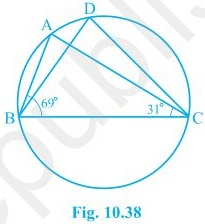∠BAC = ∠BDC (Angles in the segment of the circle)
In ΔABC,
∠BAC ∠ABC ∠ACB = 180° (Sum of the angles in a triangle)
⇒ ∠BAC + 69° + 31° = 180°
⇒ ∠BAC = 180° - 100°
⇒ ∠BAC = 80°
Thus, ∠BDC = 80°

5. In Fig. 10.39, A, B, C and D are four points on a circle. AC and BD intersect at a point E such that ∠BEC = 130° and ∠ECD = 20°. Find ∠ BAC.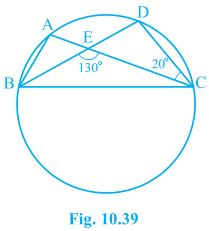∠BAC = ∠CDE (Angles in the segment of the circle)
In ΔCDE,
∠CEB = ∠CDE + ∠DCE (Exterior angles of the triangle)
⇒ 130° = ∠CDE + 20°
⇒ ∠CDE = 110°
Thus, ∠BAC = 110°

6. ABCD is a cyclic quadrilateral whose diagonals intersect at a point E. If ∠DBC = 70°, ∠BAC is 30°, find ∠BCD. Further, if AB = BC, find ∠ECD.For chord CD,
∠CBD = ∠CAD (Angles in same segment)
⇒ ∠BCD + 100° = 180°
⇒ ∠BCD = 80°
In ΔABC
AB = BC (given)
∠BCA = ∠CAB (Angles opposite to equal sides of a triangle)
∠BCA = 30°
also, ∠BCD = 80°
∠BCA + ∠ACD = 80°
⇒ 30° + ∠ACD = 80°
∠ACD = 50°
∠ECD = 50°

7. If diagonals of a cyclic quadrilateral are diameters of the circle through the vertices of the quadrilateral, prove that it is a rectangle.

Let ABCD be a cyclic quadrilateral and its diagonal AC and BD are the diameters of the circle through the vertices of the quadrilateral.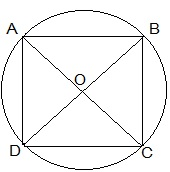∠ABC = ∠BCD = ∠CDA = ∠DAB = 90° (Angles in the semi-circle)
Thus, ABCD is a rectangle as each internal angle is 90°.

8. If the non-parallel sides of a trapezium are equal, prove that it is cyclic.

Given,
ABCD is a trapezium where non-parallel sides AD and BC are equal.
Construction,
DM and CN are perpendicular drawn on AB from D and C respectively.
To prove,
ABCD is cyclic trapezium.Proof:
In ΔDAM and ΔCBN,
∠AMD = ∠BNC (Right angles)
DM = CN (Distance between the parallel lines)
ΔDAM ≅ ΔCBN by RHS congruence condition.
Now,
∠A = ∠B by CPCT
also, ∠B + ∠C = 180° (sum of the co-interior angles)
⇒ ∠A + ∠C = 180°
Thus, ABCD is a cyclic quadrilateral as sum of the pair of opposite angles is 180°.

Page No: 186

9. Two circles intersect at two points B and C. Through B, two line segments ABD and PBQ are drawn to intersect the circles at A, D and P, Q respectively (see Fig. 10.40). Prove that ∠ACP = ∠QCD.Chords AP and DQ are joined.
For chord AP,
∠PBA = ∠ACP (Angles in the same segment) --- (i)
For chord DQ,
∠DBQ = ∠QCD (Angles in same segment) --- (ii)
ABD and PBQ are line segments intersecting at B.
∠PBA = ∠DBQ (Vertically opposite angles) --- (iii)
By the equations (i), (ii) and (iii),
∠ACP = ∠QCD

10. If circles are drawn taking two sides of a triangle as diameters, prove that the point of intersection of these circles lie on the third side.

Given,
Two circles are drawn on the sides AB and AC of the triangle ΔABC as diameters. The circles intersected at D.
Construction,
To prove,
D lies on BC. We have to prove that BDC is a straight line.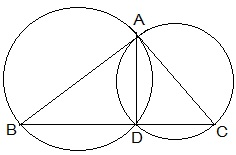Proof:
Now,
⇒ ∠BDC is straight line.
Thus, D lies on the BC.

11. ABC and ADC are two right triangles with common hypotenuse AC. Prove that ∠CAD = ∠CBD.

Given,
AC is the common hypotenuse. ∠B = ∠D = 90°.
To prove,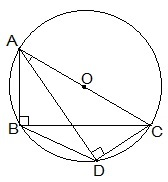Proof:
Since, ∠ABC and ∠ADC are 90°. These angles are in the semi circle. Thus, both the triangles are lying in the semi circle and AC is the diameter of the circle.
⇒ Points A, B, C and D are concyclic.
Thus, CD is the chord.
⇒ ∠CAD = ∠CBD (Angles in the same segment of the circle)

12. Prove that a cyclic parallelogram is a rectangle.

Given,
ABCD is a cyclic parallelogram.
To prove,
ABCD is rectangle.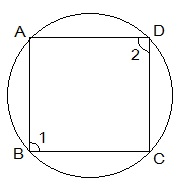Proof:
∠1 ∠2 = 180° (Opposite angles of a cyclic parallelogram)
also, Opposite angles of a cyclic parallelogram are equal.
Thus,
∠1 = ∠2
⇒ ∠1 + ∠1 = 180°
⇒ ∠1 = 90°
One of the interior angle of the paralleogram is right angled. Thus, ABCD is a rectangle.